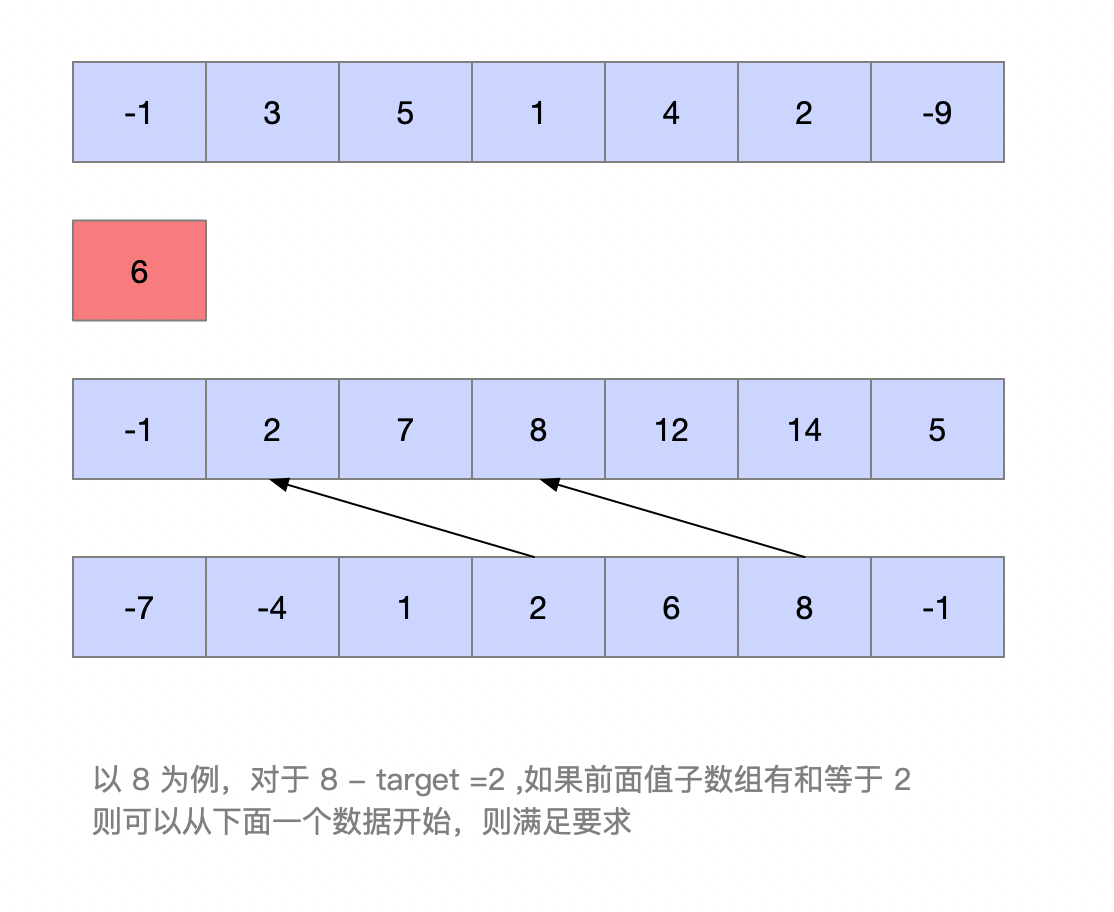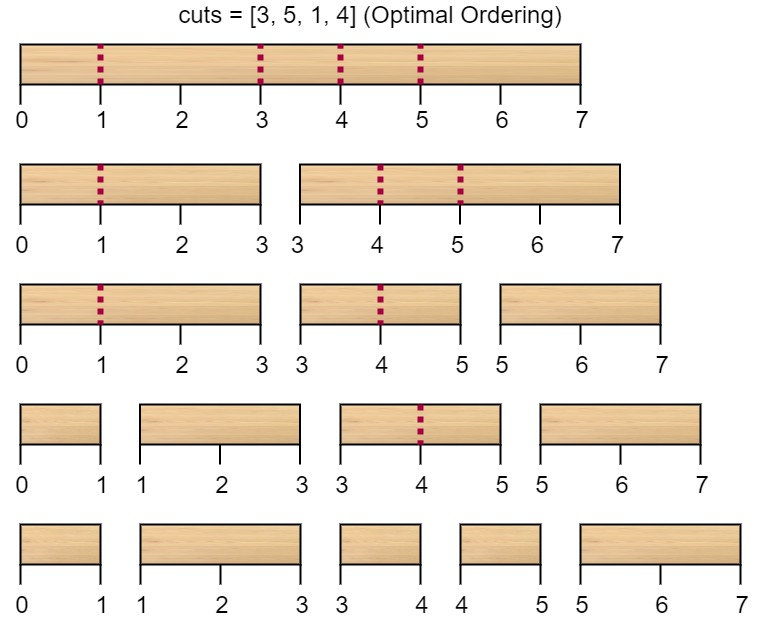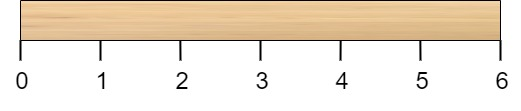#### 解答

hash+前缀

1. 首先需要理解一个问题，用 sum[0,i] 代表数组从 [0,i] 的加和，则存在 0 =< j < i < len ,sum[j,i]=sum[i]-sum[j]
2. 根据上面的理论，可以记录每一个数据的加和,即，sum[i]
3. 用当前的 sum[i] 与 `target` 去比较，如果在 sum[i]- target 的值等于 sum[j](0 =< j < i),则 arr[j:i] 是所求的数组。#### 5486. 切棍子的最小成本• `2 <= n <= 10^6`
• `1 <= cuts.length <= min(n - 1, 100)`
• `1 <= cuts[i] <= n - 1`
• `cuts` 数组中的所有整数都 互不相同
##### 解答过程

• 思路
1. 在任意一次切割时，待切割木棍的左端点要么是原始木棍的左端点 0，要么是之前某一次切割的位置；
2. 同理，待切割木棍的右端点要么是原始木棍的右端点 n，要么是之前某一次切割的位置。
3. 如果我们将切割位置数组 `cuts` 进行排序，并在左侧添加 0，右侧添加 n，那么待切割的木棍就对应着数组中一段连续的区间
4. `f[i][j]` 表示在当前待切割的木棍的左端点为 `cuts[i−1]`，右端点为 `cuts[j+1]`时,切开的成本

1. 如果当前是第一刀的话 `f[n-1]` 代表是左端点是0 ，右端点为 n 的切割成本
2. 在 0-n 一共有 n-2 切割位置，我们随机定义一个切开的位置为 `k`
3. 则第一刀的切割成本为 `cuts[n-1]-cuts` ，即是木棍的长度。
4. 木棍切开分成两段，会有两段 [0,k]和[k,n],切割位置为 [1,k-1]和【k+1,n-1】
5. 第二刀的成本和第一刀的位置相关，只有两个可能 `k-1``n-k`，对应的位置是 `f[k-1]和f[k+1][n]`
6. 我们需要寻找的是就是 `min(f[k-1],f[k+1][n])`

`f[i][j]= min(f[i][k-1],f[k+1][j])+(cuts[j+1]−cuts[i−1])`